# Unicus Olympiads - UCTO PDF Sample Papers for Class 11

Class 11 sample paper & practice questions for Unicus Critical Thinking Olympiad (UCTO) PDF Sample Papers are given below. Syllabus for UCTO is also mentioned for these exams. You can refer these sample paper & quiz for preparing for the UCTO exam.#### Resources:

##### Sample Questions from Olympiad Success:
 Q.1 Q.2 Q.3 Q.4 Q.5 Q.6 Q.7 Q.8 Q.9 Q.10
 Q.1 Determine the term that would come in place of the question mark: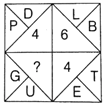a) 4 b) 6 c) 2 d) 3
 Q.2 In the question given below there are two statements followed by two conclusions numbered I and II. You have to take the two give statements to be true even if they seem to be at variance from commonly known facts and decide which of the given conclusion(s), logically follow (s) from the two given statements disregarding commonly known facts: StatementsI. Some tablets are capsules.II. All capsules are syrups. III. Some syrups are medicines.IV. All medicines are powders.Conclusions I. Some syrups are powders. II. Some syrups are tablets. a) Only conclusion I follows b) Only conclusion II follows c) Both conclusion I and II follows d) Neither conclusion I nor II follows
 Q.3 Study the following information carefully and answer the question that below. (i) 'A \$ B' means 'A is mother of B'.(ii) 'A # B' means 'A is the father of B'.(iii) 'A @ B' means 'A is the husband of B'.(iv) 'A % B' means 'A is the daughter of B'. Which of the following expression represents the relationship 'H is the brother of N'? a) N % F @ D \$ H b) N % F @ D % H c) N % F @ D \$ H # R d) H # R \$ D \$ N
 Q.4 Select the option figure which satisfies the same conditions of the placement of dots as in the question figure: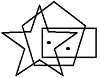a)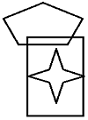b)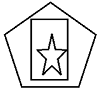c)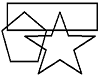d)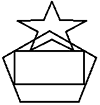Q.5 In a certain code language, TEMPORAL is written as OLDSMBSP. How is CONSIDER written in that code? a) RMNBSFEJ b) BNMRSFEJ c) RMNBJEFS d) TOPDQDCH
 Q.6 Read the following information to answer the question that follows:Seven people A, B, C, D, E, F and G are planning to enjoy boating. There are only two boats and the following are to be kept in mind.I. A will go in the same boat in which E is to go.II F cannot go in the boat in which C is unless D is also accompanying.III. Neither B nor C can be given the boat in which G is.IV. The maximum number of persons in one boat can be four only. If E gets boat with F, Which of the following is the complete and accurate list of the people who must be sitting in other boat? a) F and E b) G and A c) D and A d) C, D and B
 Q.7 Identify the region that represents students who study Biology and Computer but not Mathematics: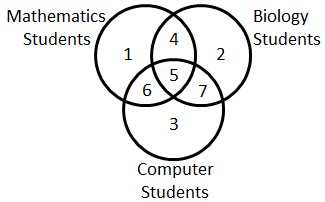a) 6 b) 2 c) 7 d) 4
 Q.8 Answer the question based on the following arrangement:R 4 P I J M Q 3 % T @ © U K 5 V 1 W \$ Y 2 B E 6 # 9 D H 8 G * Z N Four of the following five are alike in a certain way based on their positions in the given arrangement and so from a group. Which is the one that does not belong to that group? a) BE6 b) #ZG c) @©U d) 9DH
 Q.9 While facing East you turn to your left and walk 10 yards. Then, turns to your left and walk 10 yards and now turn 45o to your right and go straight to cover 50 yards. Now, in what direction are you with respect to the starting point? a) North-East b) North c) South-East d) North-West
 Q.10 If in a certain code language, 'RAM' is written as 'QSZBLN', 'LOVE' is written as 'KMNPUWDF', then how will 'ACT' be written in that language? a) ZBBDSU b) ZBBDUS c) ZBDBSU d) ZDSUBBSample PDF of Unicus Olympiads - Unicus Critical Thinking Olympiad (UCTO) PDF Sample Papers for Class 11:

 Q.1 )d Q.2 )c Q.3 )c Q.4 )b Q.5 )a Q.6 )d Q.7 )c Q.8 )b Q.9 )d Q.10 )a

Q.1 : d | Q.2 : c | Q.3 : c | Q.4 : b | Q.5 : a | Q.6 : d | Q.7 : c | Q.8 : b | Q.9 : d | Q.10 : a